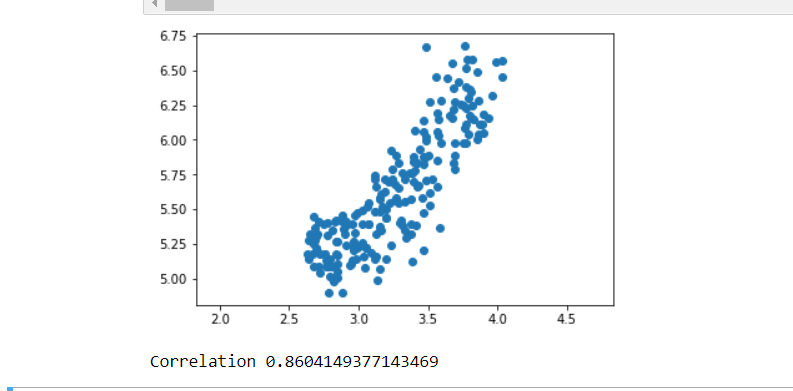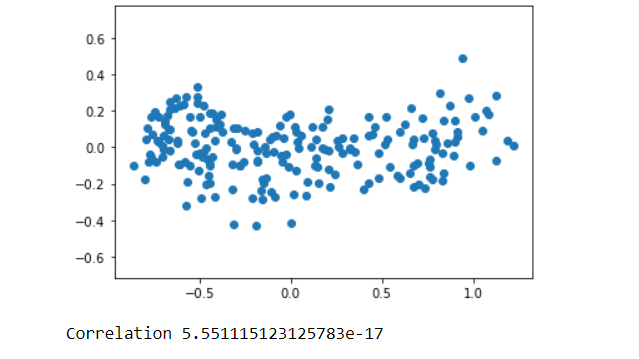# Dimension reduction | Python Unsupervised Learning -5

Read the previous post before this post.

Python Unsupervised Learning -4

# Dimension reduction

Dimension reduction finds patterns in data, and uses these patterns to  re-express it in a compressed form.  This makes subsequent computation with the data much more efficient and this can be a big deal in a world of big dataset.

### Principal Component Analysis (PCA)

PCA performs dimension reduction in two steps, and the first one, called “de-correlation” , doesn’t change the dimension of the data at all.

## Example

You can access the entire linked code above.

```import matplotlib.pyplot as plt
from scipy.stats import pearsonr

width = grains[:,0]

# Assign the 1st column of grains: length
length = grains[:,1]

# Scatter plot width vs length
plt.scatter(width,length)
plt.axis('equal')
plt.show()

# Calculate the Pearson correlation
correlation, pvalue = pearsonr(width,length)

# Display the correlation
print(correlation)``````from sklearn.decomposition import PCA

# Create PCA instance: model
model = PCA()

# Apply the fit_transform method of model to grains: pca_features
pca_features = model.fit_transform(grains)

# Assign 0th column of pca_features: xs
xs = pca_features[:,0]

# Assign 1st column of pca_features: ys
ys = pca_features[:,1]

# Scatter plot xs vs ys
plt.scatter(xs, ys)
plt.axis('equal')
plt.show()

# Calculate the Pearson correlation of xs and ys
correlation, pvalue = pearsonr(xs, ys)

# Display the correlation
print(correlation)```See you in the next article

7,422 views last month,  1 views today